What is the optimum Vsp to get the largest delta_V

expend the least energy E

and/or deliver the largest Payload?Conclusion: the same value of Vsp.

The same value of delta_V/Vsp

• maximizes speed delta_V
• minimizes energy required

Proof

Starting with...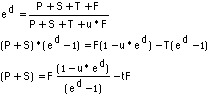And substituting...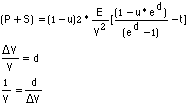Gives....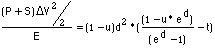Giving the left hand side to be

= [Kinetic Energy into payload and ship] / [Energy into propellant]

The right hand side is just a function Fcn (d, t, u) of three dimensionless numbers (d,t,u). Only d is related to the optimum Vsp. When the value of d is found that maximizes the function, then d=delta_V/Vsp gives the optimum Vsp.

Maximizing the term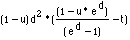which is Fcn (d, t, u), maximizes the term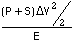. This maximizes P+S, or it maximizes delta_V, or it minimizes E.

When d = 1.59xxxx, and u and t are 0.000, this function is about .647 xxx.